# Creating Playing Cards Dynamically Using Code for Game Jams

This tutorial is different from my earlier tutorials as this one is oriented towards game jams and game prototyping, specifically card games. We are going to create a 2D playing card deck in Unity without using any art—purely with code.

## Creating Playing Cards Dynamically Using Code for Game Jams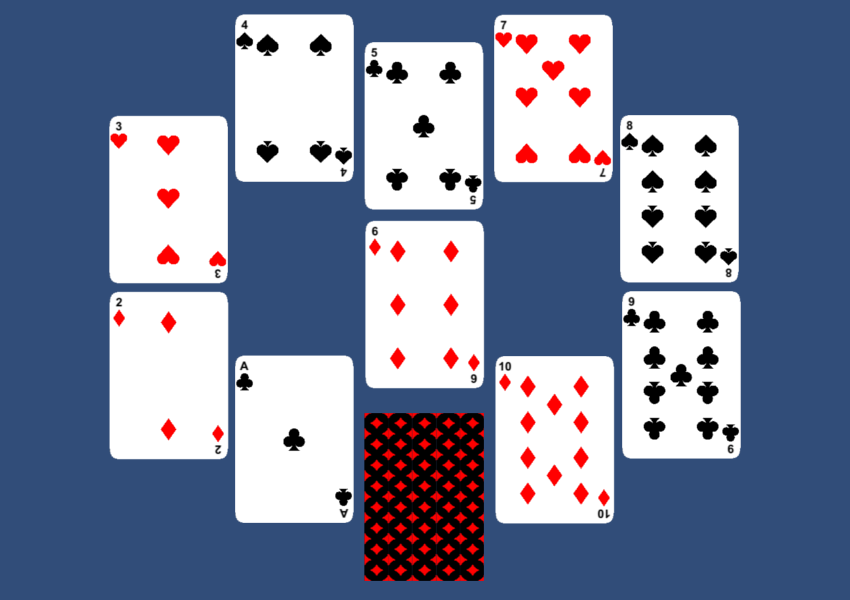## 1. Components of a Playing Card Deck

A playing card deck has a total of 52 cards with 13 cards each of 4 different symbols. In order to create one using code, we will need to create these 4 symbols, the rounded rectangular base for the card, and the design on the back of the card.

The design on the back of the card can be any abstract pattern, and there are numerous ways to create one. We will be creating a simple tileable pattern which will then be tiled to create the design. We won’t have any special design for the A, K, Q, and J cards.

## 2. Alternative Solutions

Before we start, I have to mention that there are easier solutions out there which we can use to create a deck of cards. Some of those are listed below.

1. The obvious one is to use pre-rendered art for all the designs.
2. The less obvious one is to use a font which contains all the necessary symbols. We can also turn the said font into a bitmap font to reduce draw calls and increase performance.

The font-based solution is the fastest and easiest one if you want to do quick prototypes.

## 3. Creating Textures During Runtime

The first step is to learn how to create a `Texture2D` using code which can then be used to create a `Sprite` in Unity. The following code shows the creation of a 256×256 blank texture.

``````Texture2D texture = new Texture2D(256, 256, TextureFormat.ARGB4444, false);
texture.filterMode=FilterMode.Trilinear;
texture.wrapMode=TextureWrapMode.Clamp;
texture.Apply();``````

The idea is to draw all the designs onto the texture before we use the `Apply` method. We can draw designs onto the texture pixel by pixel using the `SetPixel` method, as shown below.

``texture.SetPixel(x, y, Color.white);``

For example, if we wanted to fill out the entire texture with a color, we could use a method like this.

``````private void PaintRectangle(Texture2D texture, Rect rectBounds, Color color)
{
for (int i=(int)rectBounds.x;i<rectBounds.x+rectBounds.width;i++){
for (int j=(int)rectBounds.y;j<rectBounds.y+rectBounds.height;j++){
texture.SetPixel(i, j, color);
}
}
}
//
PaintRectangle(texture,new Rect(0,0,256,256),Color.red);``````

Once we have a `Texture2D` created, we can use it to create a `Sprite` to be displayed on screen.

``Sprite sprite = Sprite.Create(texture, new Rect(0.0f, 0.0f, texture.width, texture.height), new Vector2(0.5f, 0.5f),1);``

The complicated part in all this is the creation of the necessary designs on the texture.

## 4. Creating the Heart Shape

When it comes to the creation of the heart shape, there are many different approaches which we could use, among which are some complicated equations as well as simple mixing of shapes. We will use the mixing of shapes method as shown below, specifically the one with the triangle.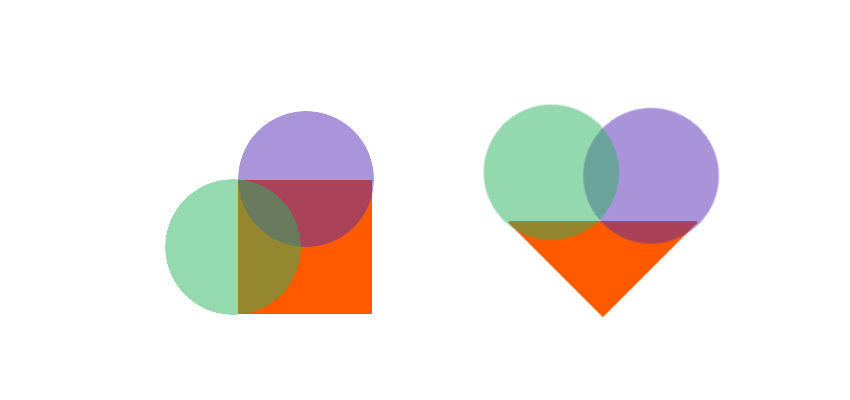As you have observed, we can use two circles and a square or a triangle to create the basic heart shape. This means it would miss those extra beautiful curves but would fit our purpose perfectly.

### Painting a Circle

Let’s brush up on some equations to paint a circle. For a circle with centre at origin and radius `r`, the equation for the point `(x,y)` on the circle is `x``2`` + y``2`` = r``2`. Now if the centre of the circle is at `(h,k)` then the equation becomes  `(x-h)``2`` + (y-k)``2`` = r``2`. So if we have a square bounding box rectangle then we can loop through all the points within that rectangle and determine which points fall inside the circle and which do not. We can easily create our `PaintCircle` method based on this understanding, as shown below.

``````private void PaintCircle(Texture2D texture,float radius, Vector2 midPoint, Color color){
Rect circleBounds=new Rect();
circleBounds.x=Mathf.Clamp(midPoint.x-(radius),0,resolution);
circleBounds.y=Mathf.Clamp(midPoint.y-(radius),0,resolution);
circleBounds.width=Mathf.Clamp(2*radius,0,resolution);
circleBounds.height=Mathf.Clamp(2*radius,0,resolution);
float iValue;
for (int i=(int)circleBounds.x;i<circleBounds.x+circleBounds.width;i++){
for (int j=(int)circleBounds.y;j<circleBounds.y+circleBounds.height;j++){
iValue=(Mathf.Sqrt(radius*radius-((j-midPoint.y)*(j-midPoint.y))));
if(i>midPoint.x-iValue&&i<midPoint.x+iValue){
texture.SetPixel(i, j, color);
}
}
}
}
PaintCircle(texture,radius,mid,Color.red);``````

Once we have the `PaintCircle` method, we can proceed to create our heart shape as shown below.

``````void PaintHearts(Texture2D texture){
//2 circles on top
float radius =resolution*0.26f;
Vector2 mid=new Vector2(radius,resolution-radius);
PaintCircle(texture,radius,mid,Color.red);
mid=new Vector2(resolution-radius,resolution-radius);
PaintCircle(texture,radius,mid,Color.red);
//triangle at bottom
float width=resolution*0.58f;
int endJ=(int)(resolution*0.65f);
int startJ=(int)(resolution*0.1f);
float delta=(width/endJ);
float midI=resolution*0.5f;
for (int i=0;i<resolution;i++){
for (int j=startJ;j<endJ;j++){
if(i>(midI-(delta*(j-startJ)))&&i<(midI+(delta*(j-startJ)))){
texture.SetPixel(i, j, Color.red);
}
}
}
}``````

The variable `resolution` is the width and height of the texture.

## 5. Creating the Diamond Shape

We will discuss two ways to draw the diamond shape.

### Painting a Simple Diamond

The easiest one is to extend the code used for the triangle and add an inverted triangle on the top to create the necessary shape, as shown below.

`````` void PaintDiamond(Texture2D texture){
float width=resolution*0.35f;
for (int i=0;i<resolution;i++){
for (int j=0;j<resolution;j++){
if(ValidDiamondPosition(i,j,width)){
texture.SetPixel(i, j, Color.red);
}
}
}
}

private bool ValidDiamondPosition(int i, int j, float width)
{
//i =col j = row
bool isValid=false;
float midI=(resolution/2.0f);
float midJ=(resolution/2.0f);
float delta=(width/midJ);
if(j>midJ){
j=resolution-j;
if(i>(midI-(delta*j))&&i<(midI+(delta*j))){
isValid=true;
}
}else{
if(i>(midI-(delta*j))&&i<(midI+(delta*j))){
isValid=true;
}
}
return isValid;
}
PaintDiamond(texture);``````

### Painting a Curvy Diamond

The second one is to use another equation to create a better, curvy version of our diamond shape. We will be using this one to create the tiling design for the back side of our card. The equation for a circle derives from the original equation of an ellipse, which is `(x/a)``2`` + (y/b)``2`` = r``2`.

This equation is the same as that of the circle when the variables `a` and `b` are both `1`. The ellipse equation can then be extended into a superellipse equation for similar shapes just by changing the power, `(x/a)``n`` + (y/b)``n`` = r``n`. So when `n` is `2` we have the ellipse, and for other values of `n` we will have different shapes, one of which is our diamond. We can use the approach used to arrive at the `PaintCircle` method to arrive at our new `PaintDiamond` method.

``````private void PaintDiamond(Texture2D texture, Rect rectBounds, Vector2 midPoint, Color color, float n=0.8f)
{
float iValue;
int a=(int)(rectBounds.width/2);
int b=(int)(rectBounds.height/2);
float nRoot=1/n;
float delta;
float partialOne;
rectBounds.width=Mathf.Clamp(rectBounds.x+rectBounds.width,0,resolution);
rectBounds.height=Mathf.Clamp(rectBounds.y+rectBounds.height,0,resolution);
rectBounds.x=Mathf.Clamp(rectBounds.x,0,resolution);
rectBounds.y=Mathf.Clamp(rectBounds.y,0,resolution);
for (int i=(int)rectBounds.x;i<rectBounds.width;i++){
for (int j=(int)rectBounds.y;j<rectBounds.height;j++){
delta=Mathf.Abs(j-midPoint.y);
partialOne=Mathf.Pow(b,n)-Mathf.Pow(delta,n);
iValue=((a/b)*Mathf.Pow(partialOne,nRoot));//+mid.x
if(i>midPoint.x-iValue && i<midPoint.x+iValue){
texture.SetPixel(i, j, color);
}
}
}
}``````

### Painting a Rounded Rectangle

The same equation can be used to create our rounded rectangle card base shape by varying the value of `n`.

``````private void PaintRoundedRectangle(Texture2D texture)
{
for (int i=0;i<resolution;i++){
for (int j=0;j<resolution;j++){
if(ValidRRPosition(i,j)){
texture.SetPixel(i, j, Color.white);
}else{
texture.SetPixel(i, j, Color.clear);
}
}
}
}

private bool ValidRRPosition(int i, float j)
{
bool isValid=false;
float iValue;
Vector2 mid;

mid=new Vector2(resolution/2,resolution/2);
int radius=(int)(resolution/2);
int a=radius;
int b=radius;
float n=16;
float nRoot=1/n;
float delta=j-mid.y;
float partialOne=Mathf.Pow(b,n)-Mathf.Pow(delta,n);
iValue=((a/b)*Mathf.Pow(partialOne,nRoot));//+mid.x
if(i>mid.x-iValue && i<mid.x+iValue){
isValid=true;
}

return isValid;
}
``````

### Painting a Tiling Design

Using this `PaintDiamond` method, we can draw five diamonds to create the tiling texture for the design on the back of our card.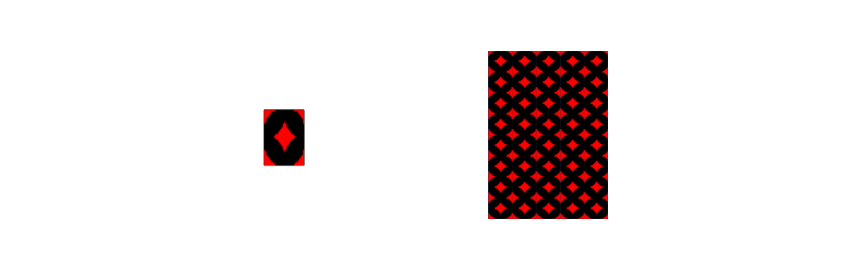The code for drawing the tiling design is as below.

`````` private void PaintTilingDesign(Texture2D texture, int tileResolution)
{
Vector2 mid=new Vector2(tileResolution/2,tileResolution/2);
float size=0.6f*tileResolution;
PaintDiamond(texture,new Rect(mid.x-size/2,mid.y-size/2,size,size),mid,Color.red);
mid=new Vector2(0,0);
PaintDiamond(texture,new Rect(mid.x-size/2,mid.y-size/2,size,size),mid,Color.red);
mid=new Vector2(tileResolution,0);
PaintDiamond(texture,new Rect(mid.x-size/2,mid.y-size/2,size,size),mid,Color.red);
mid=new Vector2(tileResolution,tileResolution);
PaintDiamond(texture,new Rect(mid.x-size/2,mid.y-size/2,size,size),mid,Color.red);
mid=new Vector2(0,tileResolution);
PaintDiamond(texture,new Rect(mid.x-size/2,mid.y-size/2,size,size),mid,Color.red);
}``````

## 6. Creating the Spades Shape

The spades shape is just the vertical flip of our heart shape along with a base shape. This base shape will be the same for the clubs shape as well. The below figure illustrates how we can use two circles to create this base shape.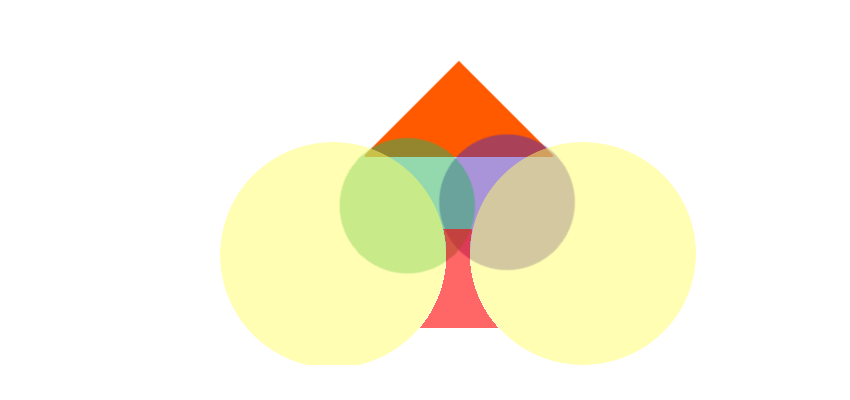The `PaintSpades` method will be as shown below.

``````void PaintSpades(Texture2D texture){
//2 circles on middle
float radius =resolution*0.26f;
Vector2 mid=new Vector2(radius,resolution-2.2f*radius);
PaintCircle(texture,radius,mid,Color.black);
mid=new Vector2(resolution-radius,resolution-2.2f*radius);
PaintCircle(texture,radius,mid,Color.black);
//triangle at top
float width=resolution*0.49f;
int startJ=(int)(resolution*0.52f);
float delta=(width/(resolution-startJ));
float midI=resolution*0.5f;
int alteredJ;
radius=resolution*0.5f;
float midJ=resolution*0.42f;
float iValue;

for (int i=0;i<resolution;i++){
//top triangle
for (int j=startJ;j<resolution;j++){
alteredJ=resolution-j;
if(i>(midI-(delta*alteredJ))&&i<(midI+(delta*alteredJ))){
texture.SetPixel(i, j, Color.black);
}
}
//bottom stalk
for (int k=0;k<resolution*0.5f;k++){
mid=new Vector2(0,midJ);
iValue=(Mathf.Sqrt(radius*radius-((k-mid.y)*(k-mid.y))));//+mid.x;
if(i>mid.x+iValue){
mid=new Vector2(resolution,midJ);
iValue=(Mathf.Sqrt(radius*radius-((k-mid.y)*(k-mid.y))));//+mid.x;
if(i<mid.x-iValue){
texture.SetPixel(i, k, Color.black);
}
}
}
}
}``````

## 7. Creating the Clubs Shape

At this point, I am sure that you can figure out how easy it has become to create the clubs shape. All we need are two circles and the base shape we created for the spades shape.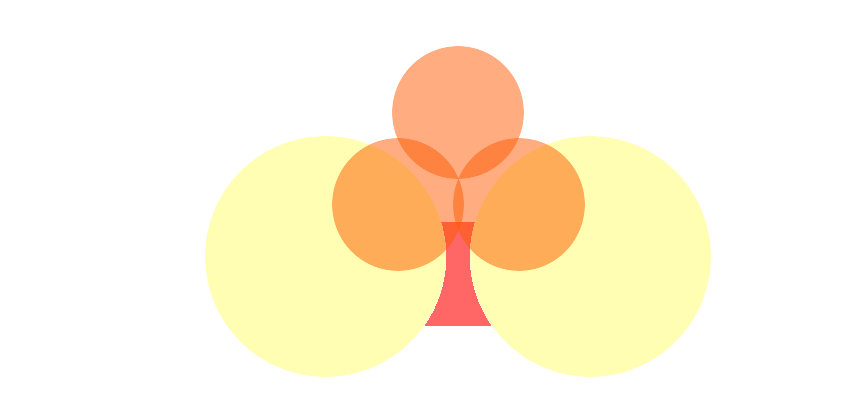The `PaintClubs` method will be as shown below.

`````` void PaintClubs(Texture2D texture){
int radius=(int)(resolution*0.24f);
//3 circles
Vector2 mid=new Vector2(resolution*0.5f,resolution-radius);
PaintCircle(texture,radius,mid,Color.black);
mid=new Vector2(resolution*0.25f,resolution-(2.5f*radius));
PaintCircle(texture,radius,mid,Color.black);
mid=new Vector2(resolution*0.75f,resolution-(2.5f*radius));
PaintCircle(texture,radius,mid,Color.black);
//base stalk
radius=(int)(resolution*0.5f);
float midY=resolution*0.42f;
int stalkHeightJ=(int)(resolution*0.65f);
float iValue;

for (int i=0;i<resolution;i++){
for (int j=0;j<stalkHeightJ;j++){
mid=new Vector2(resolution*-0.035f,midY);
iValue=(Mathf.Sqrt(radius*radius-((j-mid.y)*(j-mid.y))));//+mid.x;
if(i>mid.x+iValue){
mid=new Vector2(resolution*1.035f,midY);
iValue=(Mathf.Sqrt(radius*radius-((j-mid.y)*(j-mid.y))));//+mid.x;
if(i<mid.x-iValue){
texture.SetPixel(i, j, Color.black);
}
}
}
}
}``````

## 8. Packing Textures

If you explore the Unity source files for this project, you’ll find a `TextureManager` class which does all the heavy lifting. Once we have created all the necessary textures, the `TextureManager` class uses the `PackTextures` method to combine them into a single texture, thereby reducing the number of draw calls required when we use these shapes.

``Rect[] packedAssets=packedTexture.PackTextures(allGraphics,1);``

Using the `packedAssets` array, we can retrieve the bounding boxes of individual textures from the master texture named `packedTexture`.

``````public Rect GetTextureRectByName(string textureName){
textureName=textureName.ToLower();
int textureIndex;
Rect textureRect=new Rect(0,0,0,0);
if(textureDict.TryGetValue(textureName, out textureIndex)){
textureRect=ConvertUVToTextureCoordinates(packedAssets[textureIndex]);
}else{
Debug.Log("no such texture "+textureName);
}
return textureRect;
}
private Rect ConvertUVToTextureCoordinates(Rect rect)
{
return new Rect(rect.x*packedTexture.width,
rect.y*packedTexture.height,
rect.width*packedTexture.width,
rect.height*packedTexture.height
);
}``````

## Conclusion

With all the necessary components created, we can proceed to create our deck of cards as it is just a matter of properly laying out the shapes. We can either use the Unity UI to composite cards or we can create the cards as individual textures. You can explore the sample code to understand how I have used the first method to create card layouts.

We can follow the same method for creating any kind of dynamic art at runtime in Unity. Creating art at runtime is a performance-hungry operation, but it only needs to be done once if we save and reuse those textures efficiently. By packing the dynamically created assets into a single texture, we also gain the advantages of using a texture atlas.

Now that we have our playing card deck, let me know what games you are planning to create with it.